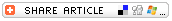## Search formBack to More Math Fun Lesson Plan
Candy Colors:
Figuring the Mean,
Median, and ModeSubjects

• Educational Technology
• Mathematics
--Algebra
--Applied Math
--Arithmetic
--Measurement
--Probability
--Statistics

• 3-5
• 6-8
• 9-12

Brief Description

Students count candy by color and then calculate mean, median, and mode.

Objectives

Students will

• learn the difference between mean, median, and mode.
• count candy and use the data to calculate mean, median, and mode (by color).
• apply their learning to another collection of data.

Keywords

math, candy, color, mean, median, mode, average, data, statistics

Lesson Plan

Before the Lesson
Provide each student with a package of colorful candy. Gummy bears, M&Ms, Skittles, Neccos, or LifeSavers will work well for this activity. Provide commercially sold candy packs or buy large quantities of candy and create your own small packs.

Activities
In this activity, students work on their own, in pairs, in small groups, in larger groups and, finally, as a whole class to figure mean, median, and mode of collections of candy data. They fill in the data they collect on the Counting Candy Colors work sheet.

Begin this lesson by modeling the process students will use to calculate and record mean, median, and mode for their candy. Then students work on their own to do the following activities:

• Count and graph the number of pieces of candy in their packs by color. Each student can graph his/her results or use the online Create-a-Graph tool to show their color-by-color tallies.
• Use the above data to figure the mean, the average number of pieces of candy of each color. For example, if the data indicates 1 red candy, 2 green candies, 3 orange candies, 7 yellow candies, and 7 purple candies, the mean, or average number of pieces of each color is 4.
1 + 2 + 3 + 7 + 7 = 20 and 20 (total candies) divided by 5 (different colors) = 4 (the average number of pieces of each color)
• Use the data to calculate the median, or middle number. For the example above (1, 2, 3, 7, 7), the median is 3.
• Use the data to calculate the mode, or the number that occurs most frequently. For the example above (1, 2, 3, 7, 7), the mode is 7.

Next, each student fills in the data on the Counting Candy Colors work sheet in the row next to Student 1. Then each pairs up with a classmate and adds that classmates data to their charts next to Student 2. Then the two students work together to figure out mean, median, and mode of their combined data and record that information on the work sheet.

This might be a good time to review the students work to be sure they have grasped the concepts of mean, median, and mode. Students might share their work, one pair at a time, with their classmates.

Next, combine two pairs of students to form groups of four. Have each student add the work sheet data for the two new people in their group next to Student 3 and Student 4. Then have them work together to figure out mean, median, and mode for all four people in the group and record the information on the work sheet.

Next, combine two groups of four and have students add the data for the four new people in their groups next to Student 5, Student 6, Student 7, and Student 8 on the worksheet. Then students work together to figure out mean, median, and mode for their combined data and record the information on the work sheet.

Finally, collect data for the entire class and challenge students to use that data to calculate mean, median, and mode for the entire class.

Assessment

Provide students with the test data for five fictional students shown below. The data shows how many answers each student got correct on a quiz of 10 questions. Instruct students to find mean, median, and mode of this data collection.

 NAME SCORE Chad 7 Karla 10 Maria 7 Steve 7 Tomas 9

Mean: 7 + 10 + 7 + 7 + 9 = 40; 40 divided by 5 = 8
Median: the middle number in this collection of data (7, 7, 7, 9, 10) is 7
Mode: the most commonly represented number in this collection of data (7, 7, 7, 9, 10) is 7

Lesson Plan Source

Education World

Submitted By

Gary Hopkins

National Standards

MATHEMATICS: Number and Operations
NM-NUM.PK-2.3 Compute Fluently and Make Reasonable Estimates
NM-NUM.3-5.3 Compute Fluently and Make Reasonable Estimates
NM-NUM.6-8.3 Compute Fluently and Make Reasonable Estimates
NM-NUM.9-12.3 Compute Fluently and Make Reasonable Estimates

MATHEMATICS: Data Analysis and Probability
NM-DATA.3-5.2 Select and Use Appropriate Statistical Methods to Analyze Data
NM-DATA.6-8.2 Select and Use Appropriate Statistical Methods to Analyze Data
NM-DATA.9-12.2 Select and Use Appropriate Statistical Methods to Analyze Data

MATHEMATICS: Representation